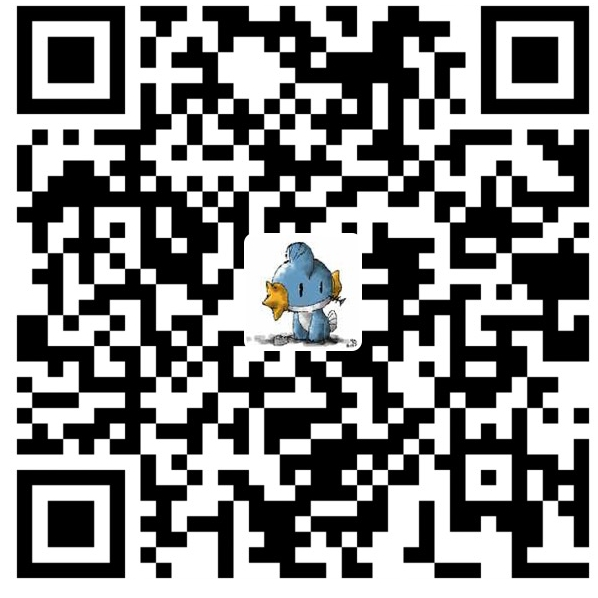# VBS也玩验证码识别```'加载图片
Set Image = CreateObject("WIA.ImageFile")
Width = Image.Width
Height = Image.Height
Set Vector = Image.ARGBData

'去除边框
For i = 1 To Vector.Count
If  (i - 1) \ Width = 0 Or _
(i - 1) \ Width = Height - 1 Or _
i Mod Width = 0 Or _
(i - 1) Mod Width = 0 Then
Vector(i) = &HFFFFFFFF
End If
Next

'去除杂点
For i = 1 To Vector.Count
If Vector(i) <> &HFF000000 Then
Vector(i) = &HFFFFFFFF
End If
Next```

```'获取横向范围
Set Range = CreateObject("WIA.Vector")
For i = 1 To Width
For j = i To Vector.Count Step Width
If Vector(j) <> &HFFFFFFFF Then
Exit For
End If
Next
Next

'写到这里肚子饿了，感谢怒云提供的算法
'先将Vector转换成String
str = Range(1)
For i = 1 To Range.Count - 1
If Range(i+1) - Range(i) < 3 Then
str = str & "," & Range(i+1)
Else
str = str & ";" & Range(i+1)
End If
Next

'然后再分组
x = Split(str,";")
For i = 0 To UBound(x)
authcode = authcode & GetCode(Split(x(i),","))
Next```

x数组保存了6个单独验证码的横向坐标，遍历数组，调用GetCode函数识别验证码。下面是函数的关键算法。

```'获取横向范围对应的纵向范围
min = 20
max = 1
For i = xn(0) To xn(UBound(xn))
For j = i To Vector.Count Step Width
If Vector(j) <> &HFFFFFFFF Then
y = (j - i) / Width
If y < min Then min = y
If y > max Then max = y
End If
Next
Next

'获取单个验证码范围内的ARGB
For i = min To max
For j = xn(0) To xn(UBound(xn))
code.Add Vector(i * Width + j)
Next
Next

'与标准的验证码对比
For Each item In Standard
Dim difference : difference = 0
For i = 1 To code.Count
'这里数组可能会越界，所以上面要On Error Resume Next
If code(i) <> Standard(item)(i-1) Then
difference = difference + 1
End If
Next
oDic(difference) = item
Next

'筛选出最匹配的
min = 80
For Each item In oDic
If item < min Then min = item
Next``````Set img = CreateObject("WIA.ImageFile")
Set v = img.ARGBData
For i = 1 To v.Count
out = out & "&H" & Hex(v(i)) & ","
Next
out = Mid(out,1,Len(out)-1)
WScript.Echo "Standard(""w"") = Array(" & out & ")" ```微信赞赏支付宝赞赏

### 24 条评论 发表在“VBS也玩验证码识别”上

1.good说道：

很有创意啊

2.micheal说道：

写得真是不错啊。。。学习了

3.郑斯彬说道：

借鉴了

4.prophetk说道：

借鉴你的代码 我还真的把西贴图片里的报价处理出来了 谢啦

5.MMMM说道：

确实不要脸。。。

6.神梦无痕说道：

图片不见了！

7.说道：

可以分享下源码吗，多谢了